## 7.2Initialisation of the k-epsilon model

Initial values and boundary conditions must be speciﬁed for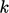and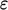to solve their respective transport equations. The ideal speciﬁcation of boundary conditions forandfollows those for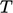described in Sec. 4.3 .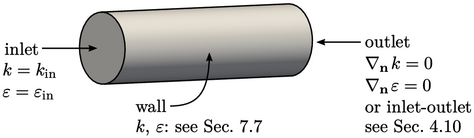Turbulence ﬁelds require: a ﬁxed value condition at inlets; zero gradient or inlet-outlet at outlets; and, a more complex speciﬁcation at solid walls, introduced in Sec. 7.7 .

Inlet values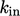and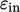must therefore be speciﬁed. There may be industry standards, published recommendations or measured data to help select these values for the speciﬁc problem being simulated.

But more often than not,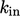andmust be estimated. Inlet and initial estimates of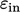are usually based on a Prandtl mixing length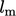from the expression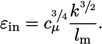(7.4)
This relation can be derived by considering a planar boundary layer. In the “log law” region (see Sec. 7.7 )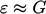which can be combined with the mixing length Eq. (6.21 ) to give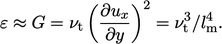(7.5)
Substituting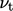from Eq. (6.31 ) yields Eq. (7.4).

A value for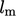must then be speciﬁed in order to calculate inlet and initial values of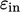from Eq. (7.4 ). Procedures to estimateare described in Sec. 7.3 .

Inlet and initial estimates for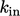can be calculated by(7.6)
from a speciﬁed turbulent intensity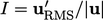, the ratio of the root-mean-square (RMS) of turbulent ﬂuctuations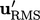to the mean ﬂow speed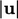. The expression is derived from the deﬁnition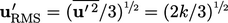.

A value for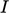must then be speciﬁed in order to calculate the inlet and initial values of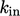from Eq. (7.6 ). Procedures to estimateare also described in Sec. 7.3 . The values of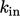and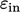at inlet boundaries inﬂuence the solution throughout the CFD simulation, so should be estimated as accurately as possible.

The accuracy of the initial (internal) values is not so critical, since they do not inﬂuence the solution beyond a short period at the beginning of a simulation.

Initial values can, however, aﬀect stability during the early steps of a CFD simulation. The ﬂow boundary conditions generally cause sudden impulses which can generate large forces, causing ﬂuctuations in the solution of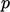. Higher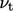, based on initial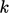and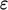values, tends to cause larger ﬂuctuations, which may make the solution of the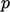-equation unstable.

Notes on CFD: General Principles - 7.2 Initialisation of the k-epsilon model